# A uniform slender rod AB rests on a friction horizontal surface, and a force P of magnitude 0.25 lb is applied at A in a direction perpendicular to the rod. Knowing that the rod weighs 1.75 lb. determine the acceleration of (a) point A, (b) point B.

Question-AnswerCategory: Engineering MechanicsA uniform slender rod AB rests on a friction horizontal surface, and a force P of magnitude 0.25 lb is applied at A in a direction perpendicular to the rod. Knowing that the rod weighs 1.75 lb. determine the acceleration of (a) point A, (b) point B.

A uniform slender rod AB rests on a friction horizontal surface, and a force P of magnitude 0.25 lb is applied at A in a direction perpendicular to the rod. Knowing that the rod weighs 1.75 lb. determine the acceleration of (a) point A, (b) point B.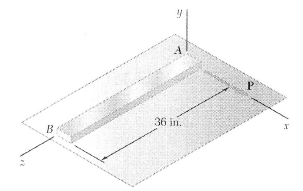Step: 1

Draw the kinematic diagram of the bar as follows:Step: 2

Calculate the mass moment of inertia of the rod about its center.Here,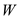is the weight of the rod,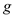is the acceleration due to gravity and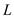is the length of the rod.
Substitute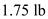for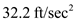forandfor.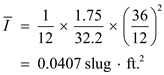Step: 3

Consider the force equilibrium equation:Substituteforandfor g.Consider the moment equilibrium condition about point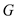.Substituteand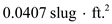for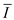.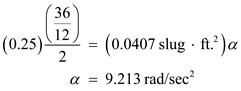Step: 4

(a)
Calculate the acceleration of point A.Substitute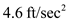forforandfor.Therefore, the acceleration of pointis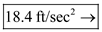.

Step: 5

(b)
Calculate the acceleration of point.Substitutefor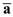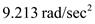for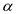and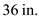for.Therefore, the acceleration of point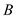is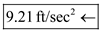.